# Data Compression with Huffman’s Algorithm

From Algorithms and Data Structures in Action by Marcello La Rocca

This article discusses Huffman’s Algorithm: what it is and what you can do with it.

_________________________________________________________________

Take 37% off Algorithms and Data Structures in Action. Just enter fccrocca into the discount code box at checkout at manning.com.
_________________________________________________________________

Huffman’s algorithm is probably the most famous data compression algorithm. You probably have already studied in your introduction to CS course. It is a simple, brilliant greedy algorithm that, despite not being the state of the art for compression anymore, was a major breakthrough in the ’50s.

A Huffman code is a tree, built bottom up, starting with the list of different characters appearing in a text and their frequency. The algorithm iteratively

1. selects and removes the two elements in the list with the smallest frequency
2. then creates a new node by combining them (summing the two frequencies)
3. and finally adds back the new node to the list.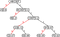Figure 1 A Huffman coding tree built from this character frequency table:A=0.6, B=0.2, C=0.07, D=0.06, E=0.05, F=0.02.

While the tree itself is not a heap, a key step of the algorithm is based on efficiently retrieving the smallest elements in the list, as well as efficiently add new elements to the list. You probably have guessed by now that, once again, that’s where heaps come to the rescue.

Let’s take a look at the algorithm itself, in listing 1.

We assume the input for the algorithm is a text, stored in a string (of course, the actual text might be stored in a file or stream, but we can always have a way to convert it to a string), and the output is a map from characters to binary sequences.

The first sub-task that we need to perform is to transform the text: we want to compute some statistics on it, to understand what are the most used and least used characters in it. To that end, we compute the frequency of characters in the text.

The details of the `ComputeFrequencies` method at line #1 are both out of scope and (at least in its basic version) simple enough, and there is no need to delve into that helper method here.

Listing 1 The Huffman Coding Algorithm

`function huffman(text)   charFrequenciesMap ← ComputeFrequencies(text)         #1   priorityQueue ← MinHeap()                             #2   for (char, frequency) in charFrequenciesMap                #3     priorityQueue.insert(TreeNode([char], frequency))        #4   while priorityQueue.size > 1                               #5     left ← priorityQueue.top()                          #6     right ← priorityQueue.top()                         #7     parent ← TreeNode(left.chars + right.chars,                       left.frequency + right.frequency)      #8     parent.left ← left                                  #9     parent.right ← right                                #10     priorityQueue.insert(parent)                             #11   return buildTable(priorityQueue.top(), [], Map())          #12`

Once we have computed the frequency map, we create a new priority queue and then at lines #3 and #4 we iterate over the frequency map, creating a new `TreeNode` for each character and then adding it to the priority queue. Obviously, considering the subject of this chapter, for the queue we use a heap, and in particular a min-heap, where the element at the top is the one with the smallest value for the priority field. And in this case the priority field is (not surprisingly) the frequency field of the `TreeNode`.

Each `TreeNode`, in fact, contains two fields (besides the pointers to its children): a set of characters, and the frequency of those characters in the text, computed as the sum of the frequencies of individual characters.

If you look at figure 1, you can see that the root of the final tree is the set of all characters in our example text, and hence the total frequency is 1.

This set is split into two groups, each of which is assigned to one of the root’s trees, and so on each internal node is similarly split till we get to leaves, each of which contains just one character.

Back to our algorithm, you can see how the tree in figure 1 is constructed bottom-up, and lines #2 to #4 in listing 1 take care of the first step, creating the leaves of the tree and adding them to the priority queue.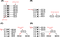Figure 2 The first step in the Huffman coding algorithm. As mentioned in the text, the algorithm will use 2 auxiliary data structures, a priority queue and a binary tree. Each tree node will have a value, a set of characters in the text, and a priority, the sum of the frequencies of those characters in the text.(A) Initially we create one tree node for each character, associated with its frequency in the text. We also add each node into a priority queue, using the frequency as its priority (smaller frequency means higher priority hence we would use a min-heap).(B) We extract two elements from the top of the priority queue.© We create a new tree node to which we add the two nodes extracted at step (B) as its children. By convention, we assume that the smallest node will be added as left child and the second-smallest as right child (but any consistent convention works here). The newly-created node will hold the union of the set of characters in its children as value, and the sum of the two priorities as priority.(D) Finally, we can add the new root for this subtree back to the priority queue.Note that the nodes in the heap are showed in sorted order, but just for the sake of simplicity: as we stated, the order in which nodes are stored inside a priority queue is an implementation detail, the contract for PQ’s API only guarantees that when we dequeue two elements, those will be the ones with the smallest frequencies.

Now, from line #5 we enter the core of the algorithm: until there is only one element left in the queue, we extract the top `TreeNode` entries, in lines #6 and #7: as you can see in figure 2.B, those two elements will be the subtrees with the lowest frequencies so far.

Let’s call this subtrees `L` and `R` (the reason for these names will be apparent soon).

Figure 2.C shows the actions performed in lines #8 to #10 of our pseudocode: a new `TreeNode` is created (let’s call it `P`) by merging the two entries’ character sets, and setting its frequency as the sum of the old subtrees’ frequencies. Then the new node and two subtrees are combined in a new subtree, where the new node `P` is the root and the subtrees `L` and `R` are its children.

Finally, at line #11 we add this new subtree back into the queue: as it’s shown in figure 2.D, it sometimes can be placed at the top of the queue, but that’s not always the case: the priority queue will take care of this detail for us (notice that here the priority queue is used as a black box)

Listing 2 The Huffman Coding Algorithm (building a table from the tree)

`function buildTable(node, sequence, charactersToSequenceMap)   if node.characters.size == 1 then                                   #1     charactersToSequenceMap[node.characters] ← sequence            #2   else                                                                #3     if node.left <> none then                                         #4       buildTable(node.left, sequence + 0, charactersToSequenceMap)    #5     if node.right <> none then                                        #6       buildTable(node.right, sequence + 1, charactersToSequenceMap)   #7   return charactersToSequenceMap                                      #8`

These steps are repeated until there is only 1 element left in the queue (figure 3 shows a few more steps), and that last element will be the `TreeNode` that is the root of the final tree.

We can then use it, in line #12, to create a compression table, which will be the final output of the `huffman` method. In turn, the compression table can be used to perform the compression of the text by translating each one of its characters into a sequence of bits.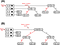Figure 3 The result of the next couple of steps in the Huffman coding algorithm:(A) We dequeue and merge the top two nodes on the heap, C and D. At the end of this step, EF and CD becomes the two smallest nodes in the heap.(B) Now we merge those two nodes into CDEF, and we add it back to the heap. Which node between CDEF and B will be kept at the top of the priority queue is an implementation detail, and it’s irrelevant for the Huffman coding algorithm (the code will change slightly depending on which one is extracted first, but it’s compression ratio will remain unchanged).The next steps are easy to figure, also using figure 1 as a reference.

While we won’t show this last step, we provide listing 2 with the steps needed to create a compression table from the tree in figure 1; while this goes beyond the scope of this chapter (since the method doesn’t use a priority queue), providing a brief explanation should help those readers interested in writing an implementation of Huffman coding.

We wrote the `buildTable` method using recursive form. As explained in appendix E, this allows us to provide cleaner and more easily understandable code, but in some languages concrete implementations can be more performant when implemented using explicit iterations.

We’re going to pass 3 arguments to the method: a `TreeNode node`, that is the current node in the traversal of the tree, a sequence, which is the path from the root to the current node (where we add a 0 for a “left turn” and a 1 for a “right turn”) and the `Map` that will hold the associations between characters and bit sequences.

At line #1, we check if the set of characters in the node has only one character. If it does, it means we have reached a leaf, and so the recursion can stop: the bit sequence that is associated with the character in the node is the path from root to current node, stored in the `sequence` variable.

Otherwise we check if the node has left and right children (it will have at least one, because it’s not a leaf) and traverse them. The crucial point here is how we build the sequence argument in the recursive calls: if we traverse the left child of the current node, we add a 0 at the end of the sequence, while if we traverse the right child, we add a 1.

Table 1 shows the compression table produced starting from the tree shown in figure 1; the last column would not be part of the actual compression table, but it’s useful to understand how most used characters end up translated into shorter sequences (which is the key to an efficient compression).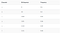Table 1 The compression table created from the Huffman tree in figure 1

Looking at the sequences, the most important property is that they form a prefix code: no sequence is the prefix of another sequence in the code.

This property is the key point for the decoding: iterating on the compressed text, we immediately know how to break it into characters.

For instance, in the compressed text `1001101`, if we start from the first character, we can immediately see the sequence `10` that matches `B`, then the next `0` matches `A`, and finally `1101` matches `D`, so the compressed bit sequence is translated into `“BAD”`.

## Performance Analysis: Finding the best Branching Factor

We have seen that priority queues are a key component in the Huffman compression pipeline; in chapter 2 of “Algorithms and Data Structures in Action”, we describe an efficient implementation of priority queues, the binary heap, and its advanced variant, the d-ary heap.

To sum it up, the difference between a binary (aka 2-ary) heap and its generalization, a d-ary heap, is the branching factor, the maximum number of children any node of the heap can have.

If we measure the performance of our heap’s methods as the number of swaps performed, we have shown that we have at most `h` swaps per-method call, if `h` is the height of the heap. This is because the heap is a complete balanced tree, the height of a d-ary heap is exactly `log`D`(n)`.

Therefore, for both methods insert and top, it would seem that the larger the branching factor, the smaller the height, and in turn the better heap performance should be.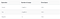Table 2 Main operations provided by a d-ary heap, and the number of swaps (assuming n elements)

But just limiting to swaps doesn’t tell us the whole story: you also have to dive into a performance analysis of these two methods, and also take into consideration the number of array accesses, or equivalently the number of comparisons on heap elements, that are needed for each method. While `insert` accesses only one element per heap’s level, method `top` traverses the tree from root to leaves, and at each level it needs to go through the list of children of a node: therefore, it needs approximately up to `D*log`D`(n)` accesses, on a heap with branching factor `D` and containing `n` elements.

Tables 2 and 3 summarize the performance analysis for the three main methods in heaps’ API.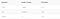Table 3 Cost of main operations provided by heaps as number of comparisons (assuming n elements)

So, for method `top`, a larger branching factor doesn’t always improve performance, because while `log`D`(n)` becomes smaller, `d` becomes larger. Bringing it to an extreme, if we choose `D > n-1`, then the heap becomes a root with a list of `n-1` children, and so `insert` will require just `1` comparison and `1` swap, while `top` method will need `n` comparisons and `1` swap (in practice, as bad as a keeping an unsorted list of elements).

There is no easy way to find a value for `D` that minimizes function `f(n) = D*log`D`(n)` for all values of `n`, and besides, these formulas give us just an estimate of the maximum number of accesses/swaps performed, the exact number of comparisons and swaps actually performed depends on the sequence of operations, and on the order in which elements are added.

Then the question arises: how do we choose the best value for the branching factor?

The best we can do here is profiling our applications to choose the best value for this parameter. In theory, applications with more calls to `insert` than to `top` will perform better with larger branching factors, while when the ratio of calls between the two methods approaches 1.0, a more balanced choice will be best.

## Profile (like there is no tomorrow)

And so, we are stuck with profiling. If you are asking yourself “what is profiling?” or “where do I start?”, here there are a few tips:

1. Profiling means measuring the running time and possibly the memory consumption of different parts of your code.
2. It can be done at a high level (measuring the calls to high level functions) or lower level, for each instruction, and although you can set it up manually (measuring the time before and after a call), there are great tools to aid you–usually guaranteeing an error-free measurement.
3. Profiling can’t give you general-purpose answers: it can measure the performance of your code on the input you provide. In turn, this means that the result of profiling is as good as the input you use, meaning that if you only use a very specific input, you might tune your code for an edge case, and it could perform poorly on different inputs. Also, another key factor is the data volume: to be statistically significant, it can be nice to collect profiling results on many runs over (pseudo)random inputs. It also means that results are not generalizable to different programming languages, or even different implementations in the same language.
4. Profiling requires time. The more in depth you go with tuning, the more time it requires. Remember Donald Knuth’s advice: “premature optimization is the root of all evil”. Meaning you should only get into profiling to optimize critical code paths: spending 2 days to shave 5 milliseconds on an application that takes 1 minute, is frankly a waste of time (and possibly, if you end up making your code more complicated to tune your app, it will also make your code worse).

If, in spite of all of the disclaimers above, you realize that your application actually needs some tuning, then brace yourself and choose the best profiling tool available for your framework.

In our example, we will profile a `Python` implementation of Huffman encoding and d-ary heap. You can check out the implementation on our repo on GitHub.

Code and tests are written in Python 3, specifically using version 3.7.4, the latest stable version at the time of writing. We are going to use a few libraries and tools to make sense of the profiling stats we collect:

To make your life easier, if you’d like to try out the code I suggest you install Anaconda distribution, that already includes latest Python distribution and all the packages above.

To do the actual profiling, we use cProfile package, which is already included in the basic `Python` distro.

We won’t explain in detail how to use `cProfile` (lots of free material online covers this, starting from Python docs linked above), but to sum it up, `cProfile` allows running a method or function and records the per-call, total, and cumulative time taken by every method involved.

Using pStats.Stats, we can retrieve and print (or process) those stats: the output of profiling looks something like what’s shown in figure 4.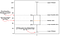Figure 5 Explaining how to read an example boxplot. Boxplots show the distribution of samples using a nice and effective chart. The main idea is that the most relevant samples are those whose values lie between the first and third quartile, i.e. we find 3 values, Q1, Q2 (aka the median), and Q3 such that:25% of the samples are smaller than Q1;50% of the samples are smaller than Q2 (which is the very definition of median, by the way);75% of the samples are smaller than Q3.The box in the boxplot is meant to clearly visualize the values for Q1 and Q3.Whiskers, instead, shows how far from the median the data extends. Additionally,, we could also display outliers, i.e. samples that are outside the whiskers’ span. Sometimes outliers, though, end up being more confusing than useful, so in this example we will not use them.

Now, to reduce the noise, we are only interested in a few methods, specifically the functions that use a heap, in particular `_frequency_table_to_heap`, which takes the dictionary with the frequency (or number of occurrences) of each character in the input text, and creates a heap with one entry per character, and `_heap_to_tree`, which in turn takes the heap created by the former function, and uses it to create the Huffman encoding tree.

We also want to track down the calls to the heap methods used: `_heapify`, `top` and `insert`. So, instead of just printing those stats, we can read them as a dictionary, and filter the entries corresponding to those five functions.

To have meaningful, reliable results, we also need to profile several calls to the method `huffman.create_encoding`, and so processing the stats and saving the result to a CSV file seems the best choice anyway.

To see the profiling in action, check out our example on GitHub. The example profiles several calls to the method creating a Huffman encoding over an ensemble of large text files and a bitmap image. The bitmap needs to be duly pre-processed in order to make it processable as text–the details of this pre-processing are not particularly interesting, but for the curious reader: we encode image bytes in base64 to have valid characters.

## Interpreting Results

Now that we stored the results of profiling in a CSV file, we just need to interpret them to understand what’s the best choice of branching factor for our Huffman encoding app.

At this point it should look like a piece of cake, right?

We can do it in many ways, but my personal favorite is displaying some plots in a Jupyter notebook: take a look here at an example–isn’t it great that GitHub lets you already display the notebook without having to run it locally?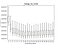Figure 6 The distribution of running times for the two high level functions in huffman.py using a heap:(above): mean running time by branching factor.(below): boxplots for the distribution of running times for each branching factor. All charts are created using data about the running time per single call, for the compression of an ensemble of text files.

Before delving into the results, though, we need to take a step back: since we will use a boxplot to display our data, let’s make sure we know how to interpret these plots first: if you feel you could use a hint, figure 5 comes to the rescue!

To make sense of the data our example notebook uses Pandas library, with which we read the CSV file with the stats and transform it into a `DataFrame`, an internal Pandas representation that you can think of as a SQL table on steroids: in fact, using this `DataFrame` we can partition data by test case (image versus text), then group it by method, and finally process each method separately and display results, for each method, grouped by branching factor. Figure 6 shows these results for encoding a large text, comparing the two main functions in Huffman encoding that use a heap.

If we look at those results, we can confirm a few points:

• 2 is never the best choice for a branching factor;
• A branching factor of 4 or 5 seems a good compromise;
• There is a consistent difference (even -50% for `_frequency_table_to_heap`) between a binary heap and the d-ary heap with best branching factor.

There is, however, also something that might look surprising: `_frequency_table_to_heap` seems to improve with larger branching factors, while `_heap_to_tree` has a minimum for branching factors around 9 and 10.

As you can see, the former method only calls the`_push_down` helper method, while the latter uses both `top` (which in turn calls `_push_down`) and `insert` (relying on `_bubble_up` instead), so we would expect to see the opposite result. It is true, in any case, that even `_heap_to_tree` has a ratio of 2 calls to `top` per `insert`.

To double check that this result is not biased because of the choice of texts, let’s take a look at the running times of encoding a bitmap image (figure 7).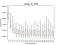Figure 7 The distribution of recorded running times for the two high level functions in huffman.py using a heap: mean running time by branching factor on top and boxplots for the distribution of running times for each branching factor below. All charts are created using data about the running time per single call, for the compression of a single bitmap image, encoded in base64.

The result looks somehow similar, making input bias less likely.

We should then go back to the text test-case: let’s then delve into the internals of these high-level methods, and see the running time per-call of the heap’s internal method `_heapify` (figure 9), of the API’s methods `top` and `insert` and their helper methods `_push_down` and `_bubble_up` (figure 8 and 10).

Before digging into the most interesting part, let’s quickly check out method insert: figure 10 shows that there are no surprises here, the method tends to improve with larger branching factors, as does `_bubble_up`, its main helper.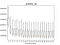Figure 8 The distribution of recorded running times per-call for insert and _bubble_up.

Method `_heapify`, instead, shows a trend similar to `_frequency_table_to_heap` – as expected, since all this method does is to create a heap from the frequency table. Still, is it a bit surprising that _`heapify`‘s running time doesn’t degrade with larger branching factors?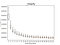Figure 9 The distribution of recorded running times per-call for heapify.

Now to the juicy part: let’s take a look at `top`, in figure 11. If we look at the running time per-call, the median and distribution clearly show a local minimum (around `D==9`), as we would have expected considering that the method’s running time is `O(D*log`D`(n))`, as we have discussed above and summarized in table 2. Not surprisingly, the `_push_down` helper method has an identical distribution.

If we look at listings 2.7 and 2.4, sections 2.6.2 and 2.6.4 of Algorithms and Data Structrures in Action, it’s clear how `pushDown` is the hearth of method `top`, and in turn the largest chunk of work for the latter is the method that at each heap level retrieves the child of the current node, we called it `highestPriorityChild`.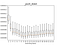Figure 10 The distribution of recorded running times per-call for top and _push_down.

And if we then look at the running time per-call for `_highest_priority_child` (figure 11), we have a confirmation that our profiling does make sense, as the running time per-call increases with the branching factor: the largest `D` is, the longest list of children for each node, which this method need to traverse entirely in order to find which tree branch should be traversed next.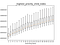Figure 11 The distribution of recorded running times per-call for _highest_priority_child.

You might then wonder: why then `_push_down` doesn’t have the same trend? Remember that while the running time for `_highest_priority_child` is `O(D)`, in particular with `D` comparisons, `_push_down` performs (at most) `log`D`(n)` swap sand `D*log`D`(n)` comparisons, because it calls `_highest_priority_child` at most `D` times.

The largest it is the branching factor `D`, the fewer calls are made to `_highest_priority_child`: this becomes apparent if, instead of plotting the running time per-call for `_highest_priority_child`, we use the total cumulative time (the sum of all calls to each method), as shown in figure 12: there we can see again how this composite function, `f(D) = D*log`D`(n)`, has a minimum at `D==9`.

## The Mystery with Heapify

So, summing up, `_heapify` keeps improving even at larger branching factors, although we can also say it practically plateaus after `D==13`, but this behavior is not caused by methods `top` and `_push_down`, which do behave as expected.

There could be a few explanations for how `_heapify` running time grows:

1. It’s possible that, checking larger branching factors, we discover that there is a minimum (we just haven’t found it yet).
2. The performance of this method heavily depends on the order of insertions: with sorted data it performs way better than random data.
3. Implementation-specific details makes the contribution of calls to `_push_down` less relevant over the total running time.

But… are we sure that this is in contrast with theory?

You should know by now that I like rhetorical questions: this means it is time to get into some Math.

And indeed, if we take a closer look at section 2.6.7 on the book, we can find out that the number of swaps performed by `_heapify` is limited by:

As you can imagine, analyzing this function is not straightforward. But, and here is the fun part, now you can certainly plot the function using your (possibly newly acquired) Jupyter Notebook skills; turns out that, indeed, plotting this function of `D` for a few values of `n`, we get the charts in figure 13, showing that, despite the single calls to `_push_down` will be slower with a higher branching factor, the total number of swaps performed by `_heapify` is expected to decrease.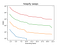Figure 13 The upper bound for the number of swaps needed by method heapify, as a function of the branching factor D. Each line is a different value of n. Notice that the y axe uses a logarithmic scale.

So, mystery solved, `_heapify` does behave as expected–and good news too, it gets faster as we increase the running time.

## Choosing the Best Branching Factor

For a mystery solved, there is still a big question standing: what is the best branching factor to speed up our Huffman encoding method? We digressed, delving into the details of the analysis of heaps, and somehow left the most important question behind.

To answer this question, there is only one way: looking at figure 14, showing the running time for the main method of our Huffman encoding library.

It shows three interesting facts:

1. The best choice seems to be `D==9`;
2. Choosing any value larger than 7 will likely be as good as;
3. While the max gain for `_frequency_table_to_heap` is 50%, and for `_heapify` even up to 80%, we merely get to a 5% here.

Notice how the chart for create_encoding looks more similar to method `_heap_to_tree` than to `_frequency_table_to_heap`: also considering point #3 above, the explanation is that the operation on the heap only contributes to a fraction of the running time, while the most demanding methods needs to be searched elsewhere (hint: for `create_frequency_table` running time depends on the length of the input file, while for the other methods it only depends on the size of the alphabet…).

You can check out the full results, including the other examples, on the notebook on GitHub. Keep in mind that this analysis uses a limited input, and as such is likely to be biased: this is just a starting point, I highly encourage you to try running a more thorough profiling using more runs on different inputs.

You can also pull the full profiling and visualization code, and delve even deeper into the analysis.

To wrap up, I’d like to highlight a few takeaways:

1. D-ary heaps are faster than binary ones. Make sure to take a look at chapter 2 of Algorithms and Data Structures in Action to learn how and why.
2. If you have parametric code, profiling is the way to tune your parameter: the best choice depends on your implementation as well as on input;
3. Be sure to run your profiling on a representative sample of your domain: if you use a limited subset, you will likely optimize for an edge case, and your results will be biased;
4. As a general golden rule, make sure to optimize the right thing: only profile critical code, perform high-level profiling first to spot the critical section of code whose optimization would give you the largest improvement, and that this improvement is worth the time you’ll spend on it.

That’s all for now. If you want to learn more about the book, check it out on liveBook here and see this slide deck.

 Greedy algorithms are characterized by their strategy of making the local-optimum choice at each step; for some problems (including the Huffman optimal encoding) this leads to the global optimal solution, although this is not the case for the majority of problems: therefore, greedy algorithms are often used as heuristics that can provide a fast but approximate solution to problems.

 Additional challenges can occur if the text is so large that either can’t be contained in memory, or where a map-reduce approach is advisable, we encapsulate all that complexity in the ComputeFrequencies method.

 Instead of the actual frequency it might be easier, and equivalent for the algorithm, just counting the number of occurrences of each character.

 It’s simply going to be a 1:1 mapping over the characters in the text, with extra attention needed to efficiently construct the bits encoding in output.

 Where D is the branching factor for the d-ary heap under analysis.

 It is, obviously, possible to find a formula for f(D)’s minima using calculus, and in particular computing the first and second order derivatives of the function.